Equipotential lines

Equipotential lines provide a quantitative way of viewing the electric potential in two dimensions. Every point on a given line is at the same potential. Such maps can be thought as topographic maps.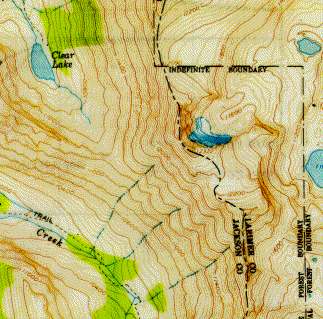For instance consider the map of the Rawah Wilderness on the right. All points on the same line are at the same elevation, just as all points on the same equipotential lines are at the same voltage. Water will always flow downhill,hence the rivers are always perpendicular to the lines on the topographic map, similar to the way electric field lines are always perpendicular to equipotential lines. When lines are close together, the slope is steep, e.g. a cliff, just as close equipotential lines indicate a strong electric field. Lakes are at the same elevation, in the same way conductors are at the same potential.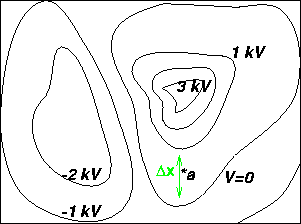The figure to the left shows equipotential lines. The electric field at point a can be found by calculating the slope at a: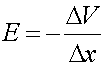where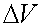is the voltage difference between the two lines near a, and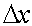is the distance between the two lines nearest a.

Rules for equipotential lines:

1. Electric field lines are perpendicular to the equipotential lines, and point "downhill".
2. A conductor forms an equipotential surface.
3. When lines are close to each other, the electric field is strong.